﻿ State Space Representation of Dynamical Systems

### State Space Representation of Dynamical Systems

Ľubica Miková, Alexander Gmiterko, Darina Hroncová

American Journal of Mechanical Engineering

## State Space Representation of Dynamical Systems

Ľubica Miková1,, Alexander Gmiterko1, Darina Hroncová1

1Department of Mechatronics, Faculty of Mechanical Engineering, Technical University of Košice, Košice, Slovakia

### Abstract

This article deals with the modeling of dynamical system in state-space representation. The state-space representation is a mathematical model of a physical system with the input, output and state variables composed by first-order differential equations. The state-space representation gives a suitable and compact way to model and analyze systems with multiple inputs and outputs. The article described the methodology for production of state of simple models of mechanical systems.

• Ľubica Miková, Alexander Gmiterko, Darina Hroncová. State Space Representation of Dynamical Systems. American Journal of Mechanical Engineering. Vol. 4, No. 7, 2016, pp 385-389. http://pubs.sciepub.com/ajme/4/7/28
• Miková, Ľubica, Alexander Gmiterko, and Darina Hroncová. "State Space Representation of Dynamical Systems." American Journal of Mechanical Engineering 4.7 (2016): 385-389.
• Miková, Ľ. , Gmiterko, A. , & Hroncová, D. (2016). State Space Representation of Dynamical Systems. American Journal of Mechanical Engineering, 4(7), 385-389.
• Miková, Ľubica, Alexander Gmiterko, and Darina Hroncová. "State Space Representation of Dynamical Systems." American Journal of Mechanical Engineering 4, no. 7 (2016): 385-389.

 Import into BibTeX Import into EndNote Import into RefMan Import into RefWorks

123
Prev Next

### 1. Introduction

Modeling of dynamic systems in practice is carried out in order to verify and solving real problems without the need to implement them. Modeling goal is not to create models with all their details, but introducing various simplifications so that the behavior of the model approached the behavior of the entire dynamic system.

To express quantitative characteristics we used the mathematical model. This model is an abstract concept of the actual process and system behavior.

For the needs of the design and verification management system is used primarily by two approaches and mathematical modeling using the so-called transfer functions and state space.

Modeling systems there are a multitude of programs that allow you to confirm the proposed simulation model without the need to actually compile it. Among the best known means include Matlab/Simulink, which provides full support not only for modeling but also for management and simulation of the proposed system [1, 2].

### 2. State-space Representation of Continuous Dynamical System

Transfer function expresses the relationship between the output and the input of the system. The main advantage of this disclosure is to obtain the equation of which can easily determine the behavior of the system.

State space describes a system using conditions and their changes over time. Výstupné veličiny (Figure 1) sústavy y(t) sú funkciou stavových x(t) a vstupných veličín u(t). The output variables (Figure 1) of the system are the function of the state values and input values. Vector of output of the model are the state matrix, which indicate the relationship between the variables describing the behavior of the system.

In this description, it is possible to observe the state of the system at the time, which sometimes allows estimating the behavior the next time step.

Under the effect of input variables u1, u2,...., um is the state vector u(t), the output signal is determined through the response of the system thru output vector y(t) and indicates the status of the state vector x(t).

If we have a system according the m inputs and r outputs, we may describe a system of n first order differential equations.

It is described by state equation: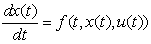(1)

where initial conditions x(0)=xS, output values of system are the function of state variables and input values. The output equation of the system is in form: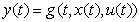(2)

where:

xT = (x1,x2,...., xn) - vector of state variables,

uT = (u1, u2,...., um) – vector of input variables,

yT = (y1, y2, ..., yr) - vector of output variables,

fT = (f1, f2,...., fn)– nonlinear vector functions,

n – number of state variables,

m – number of input,

r – number of output.

The equation (1) is called the equation of dynamics, the state equation of the system and the equation (2) is called the equation of output of the system.

These two equations describe the continuous nonlinear time variant system.

If the functions f and g are not dependend explicitly on the time t, the system description is obtained in the form: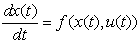(3)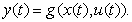(4)

These are the time invariant systems, their characteristics do not change in time.

### 3. Linear Time-invariant State Space

We described the system through state equation and output equation in the form: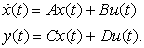(5)

With the initial condition x(0)=xS, where in the dimensions of the vector values are the same as in the two foregoing equations, and where: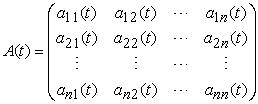(6)

is the matrix (n x n) of the system or state matrix,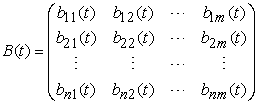(7)

is the input matrix (n x m) of the system,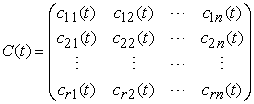(8)

is the weight matrix (r x n) of the system, is the output matrix,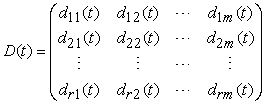(9)

is the weight matrix (r x m) of the input, je to matica prevodu, matica pôsobenia vstupu na výstup.

If the entries of matrices A, B, C, D (or in the case of at least some of them) are the time variables are the equation describing the linear time-variant (t variant, non-stationary) system. In case all of these elements are constants and the entries of A, B, C, D are matrices of constants it is the linear time-invariant (t-invariant, stationary) system.

### 4. Method of State Space

It is a method which is a graphical-analytical method for the investigation of nonlinear dynamical systems. The essence of the method is the description of the behavior of dynamic systems using illustrative geometric patterns that we call the state trajectory. When becoming acquainted with the basic concepts of state space is based on the equation of state of a nonlinear dynamical system.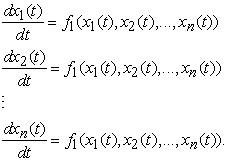(10)

Instantaneous state of a dynamic system and other behavior considered as clearly defined if at some point in time t=ti the known value of the n state variables xi (i = 1, n). These values can be considered as the coordinates of the point (x1, x2, ..., xn) of n-dimensional space, which is called the state space.

Point with coordinates x1,x2...xn representing a curve point, after which point to the change of status call state trajectory. The state trajectory in three-dimensional state space is shown in Figure 1.Download asVeiw figureFigures index
Figure 1. Determining the notion of the state trajectory

To specific initial conditions x1(0), x2(0),...xn(0) corresponds to one solution of the dynamic system. This means that each group of initial conditions corresponding to only one start point M0 and one status trajectory. Corresponds to the set of group of initial conditions corresponding to the whole set of state trajectory, which we call the phase portrait of a dynamical system. The state trajectory set really gives you an idea about the behavior of dynamic systems in time.

State-space method is best suited for the analysis of a dynamic system of the second order, because in the this case the state trajectory in the plane s.

This method is simple and opinions shall form the dynamic system of 2nd order. In this case, the state space is represented by a plane state trajectories are in the plane of the curve. State trajectory can be obtained directly from the equation of state without having to look for their solutions. State trajectory equation so we can get and we do not know the given system of equations of state directly addressed.

Suppose dynamic 2nd order system is described by nonlinear differential equations: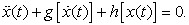(11)

Substitution: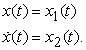(12)

Then we get a system of two equations of state: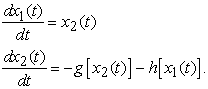(13)

If in the system (13) the second equation divided by the first equation by we get: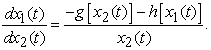(14)

The solution to equation (14) can be obtained directly in the path of the equation of the state variable x1(t) and x2(t) e.g. at state space (in this case, in the state plane). Basically solve equation (14) may not be easier than solving the original equation (11). However, equation (14) is of fundamental importance in the state space, and the method is used to study properties of solutions, for reflection on the stability etc.

### 5. Solving Selected Problems of Dynamical Systems through State Space Description

5.1. Mechanical Harmonic Oscillator

Consider a mechanical system, which forms the body of mass m secured by a spring of stiffness k to the rigid frame. The system performs linear movement along the axis of the spring (Figure 2).Download asVeiw figureFigures index
Figure 2. Mechanical harmonic oscillator

In computation it is based on the second order differential equation: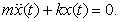(15)

After the introduction of substitutions we obtain two first-order differential equations:(16)

In matrices form: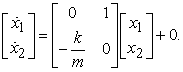(17)

Development of the displacement y dependent on x is shown in Figure 3, phase trajectory named.Download asVeiw figureFigures index
Figure 3. Phase trajectoryDownload asVeiw figureFigures index
Figure 4. The graph of the displacement of the mechanical system harmonic oscillator dependent on time

At numerical solution of differential equations using Matlab predefined functions, in which are different methods of integration. Addressing these kinematics variables is done after starting the following M-file:

function dx=funkciaHP(t,x)

m=7.5;k=50;

dx=[x(2);-k*x(1)/m];

[t,x]=ode45('funkciaHP',[0 10],[0.3; 0]);

plot(t,x)

grid on;

xlabel('t[s]');

ylabel('x[m]','dx/dt[m/s]');

legend('x','dx/dt');

5.2. Mass Spring Damper System

It is considered a mechanical system consisting of a mass m attached by a spring with stiffness coefficient k and by damper with damping coefficient b to the rigid frame. The system performs linear movement along the axis x:Download asVeiw figureFigures index
Figure 5. Mass spring damper mechanical system

This computation it is based on the second order differential equations: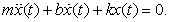(18)

After the introduction of substitutions we obtained two first-order differential equations in form: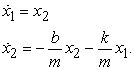(19)

In matrices form: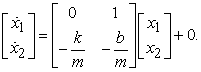(20)

Development of the displacement y dependent on x is shown in Figure 6.Download asVeiw figureFigures index
Figure 6. Phase trajectory

Development of the displacement dependent on time is shown in Figure 7.Download asVeiw figureFigures index
Figure 7. The graph of the displacement of the mechanical system
5.3. Mechanical System Consisting of Two Masses

It is considered a mechanical system shown in Figure 8, consisting of two bodies of weight m1 and m2, springs with stiffness k1, k2 and k3.Download asVeiw figureFigures index
Figure 8. Model of the mechanical system

The mechanical system is expressed in the form of the equations of motion: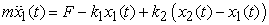(21)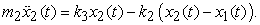(22)

State equation: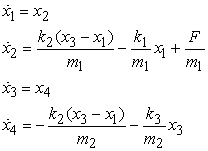(23)

State equation in matrices form: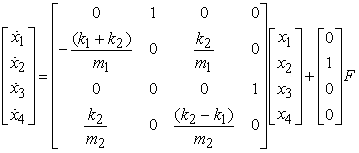(24)

Development of the displacement and of the velocity dependent on time is shown in Figure 9.Download asVeiw figureFigures index
Figure 9. The graph of the displacement and velocity of the mechanical system

### 6. Conclusion

In the state space theory is the variable vector x considered as an abstract quantity which can not generally be measured directly. The vectors of the input quantities u and output quantities y are on the other hand, having particular physical significance. Equation (5) is understood as an abstract model of the physical dependencies between physical quantities u and y and abstract variables x. To assume that at any time we chose an arbitrary but fixed coordinate system in vector space. The symbol x(t)=[x1(t), x2(t), … xn(t)] can be interpreted as a n numbers representing the coordinates of the state vector. The choice of the coordinate system in the state space is quite arbitrary, while the vectors u and y are precisely defined by its components, with the total of particular significance.

### Acknowledgements

This contribution is also the result of the project implementation: Centre for research of control of technical, environmental and human risks for permanent development of production and products in mechanical engineering (ITMS:26220120060) supported by the Research & Development Operational Program funded by the ERDF and VEGA 1/0872/16 Research of synthetic and biological inspired locomotion of mechatronic systems in rugged terrain, grant projects VEGA 1/0731/16 Development of advanced numerical and experimental methods for the analysis of mechanical systems, project KEGA 048TUKE-4/2014 Increasing of knowledge base of students in area of application of embedded systems in mechatronic systems and project KEGA 054TUKE-4/2014 The use of advanced numerical methods of mechanics as the basis for constructing the scientific development of the knowledge base of students second and third degree university study.

### References

  J. Balátě, Automatické řízení, BEN – technická literature, 1. vyd. Praha, Česko, 2003.In article  Mikleš, J.; Fikar, M., Process Modelling, Identification and Control, Springer Heidelberg New York, Berlin.In article  Ertl L., Modelling and control design of mechatronics systems on state-space, Faculty of mechanical engineering institute of solid mechanics, mechatronics and biomechanics, VUT Brno, Česko, 2009.In article  Březina, L., Stavové modely a stavové řízení , VUT Brno, Česko, 2009.In article  Schlegel, M., Goubej, M., Königskmarková, J.: Active vibration control of two-mass flexible systém using parametric Jordan form assignment. IFAC Conference on Advances in PID Control, Brescia, 2012.In article View Article